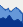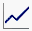• Define your upper bound to be the standard deviation within an age group added to the mean of that age group.
 • Define the lower bound to be the standard deviation within an age group subtracted from the mean of that age group.
 1 Select Help > Sample Data Library and open Big Class.jmp.
 2 Select Graph > Graph Builder.
 3 Right-click the column height and select Formula.
 4 Select Statistical > Col Mean.
 5 In the formula, click height so that the blue box is around only the variable name.
Figure 3.22 Select the height Variable
 6
 7 In the formula, select the outer box and click the plus sign.
Figure 3.23 Click the Plus Sign
 8 Click Statistical > Col Std Dev.
 9 Click height.
 10
Figure 3.24 Formula for Upper Bound
 11
 12 Click OK.
 13 In the Variables list, right-click the Transform[height] column and select Rename.
 14 Type upper and click OK.
 1 In the Variables list, right-click the column height and select Formula.
 2 In the formula, right-click on height and select Paste.
 3 Select the box around Col Std Dev (height, age) and click the +/- sign.
Figure 3.25 Selecting Col Std Dev and Making It Negative
Figure 3.26 Formula for Lower Bound
 4 Click OK.
 5 In the Variables list, right-click the Transform[height] column and select Rename.
 6 Type lower and click OK.
 1 Select age and drag it to the X zone.
 2 Select height, upper, and lower and drag them to the Y zone.
 3 Click the Area element4
 5
Figure 3.27 Area Plot
 6 Press Ctrl and drag the Line element, into the graph.
 7 In the Line options, click the triangle next to Variables. De-select Y upper and Y lower. See Figure 3.28.
Figure 3.28 Area Plot with Mean Line
 8 (Optional) Click Done.
The dark blue line connects the mean heights for each age group. As expected, height tends to increase as age increases. The shaded area shows a range of one standard deviation above and one standard deviation below the mean height at each age group.

Help created on 7/12/2018